# Determining Linear Functions

in STEMGeeks10 months ago (edited)

Hi. In this math post, I go over determining linear functions from a table of values.Pixabay Image Source

## Topics

• The Slope-Intercept Form Of A Line
• Common Difference Of Numbers As Slope
• Examples In Finding The Slope & Y-Intercept For The Equation Of A Line

## The Slope-Intercept Form Of A Line

When you have two points, a line can be created that passes these two points. On the Cartesian plane a line has a slope and a y-intercept. When x = 0 the corresponding y-value with x = 0 is the y-intercept. The equation of the line is of the form: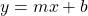where `m` is the slope of the line and `b` is the y-intercept.Pixabay Image Source

## Common Difference Of Numbers As Slope

The slope of a line can be viewed as rise over run. This rise over run is the change in y-values divided by change in x-values.

From a table a values the slope is also the common difference.

Example

xy = x + 1
-2-1
-10
01
12
23

From the above example, the slope of the line is just 1. As x increases by 1, the value of y increases by 1 each time. The slope would be 1 divided by 1 which is just 1. This value of 1 is the slope which is also the difference in y-values as x increases by 1 each time.

Example Two

Determine the equation of the line from the following table.

xy
0-2
11
24
37
410

From here the value of `y` goes up by 3 for each 1 unit increase of `x`. This value of 3 is the slope for the liine that passes the `(x, y)` points from the table of values.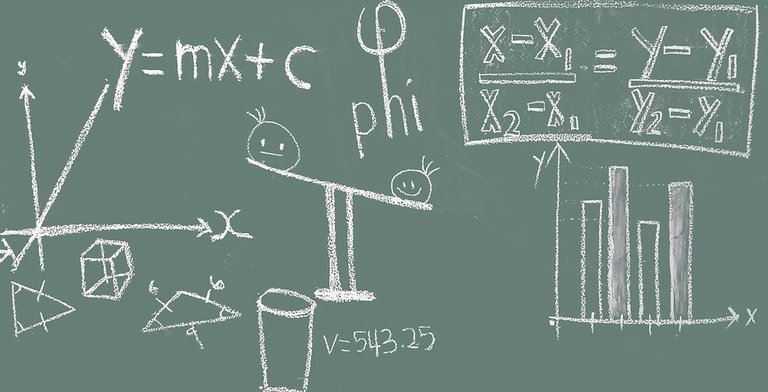Pixabay Image Source

## Examples In Finding The Slope & Y-Intercept

There are cases where a y-intercept is not given from a table of values. Let's look at some examples.

Example One

xy
2-3
40
63
86

In this table of values we do not have a y-intercept (y-value when x = 0). For every increase of `x` by 2 we increase `y` by 3. This is a slope of 3 divided by 2.

So far we have the equation of a line as: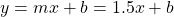To find the y-intercept, we can use a (x, y) pair from the table of values to help solve for the y-intercept represented by `b`. I will use the point `(4, 0)` as my `(x, y)` point.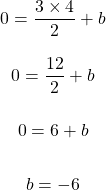The equation of the line for the table of values in this example is `y = 1.5x - 6`.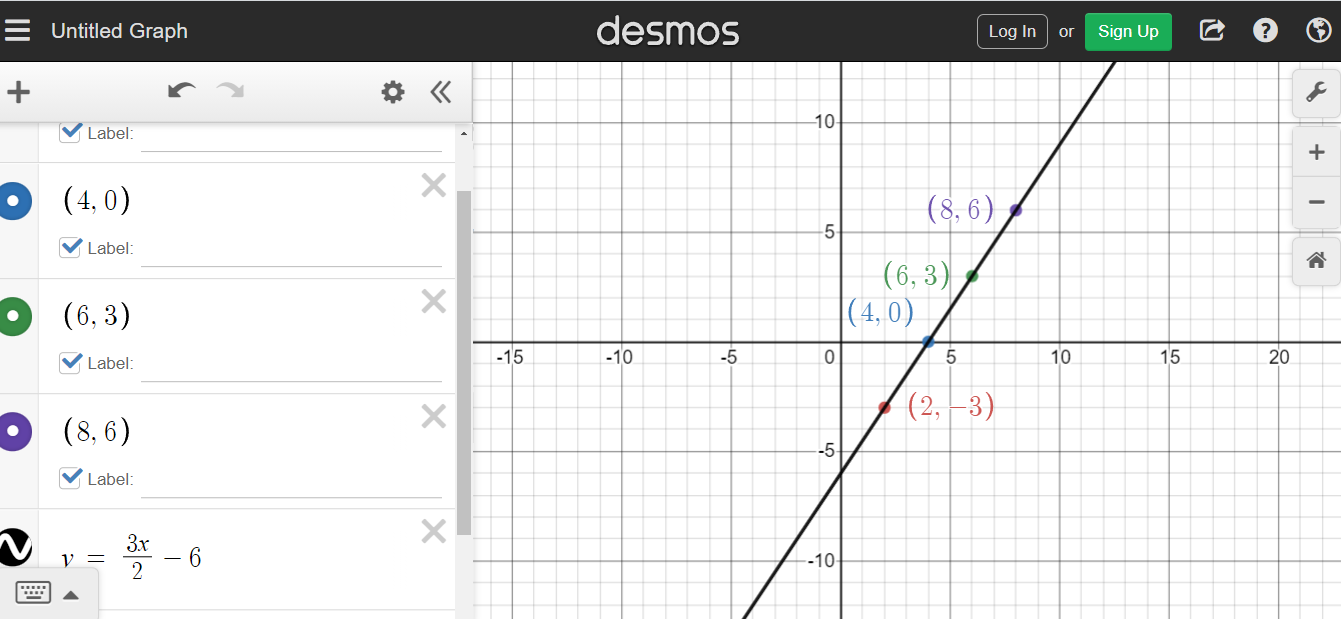Desmos Screenshot

Example Two

xy
18
42
50
10-10

This table of values does not have equal spacing with the x-values. Do be mindful of this and check that the slope is the same between any two points here. For the purpose of this exercise I have made the table of values such that the slope is the same between any two points.

For computing the slope I use the points `(4, 2)` and `(5, 0)`.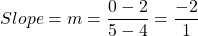Now we solve for `b` in `y = mx + b`. The point `(1, 8)` is used for `(x, y)` to find `b`.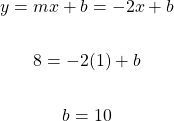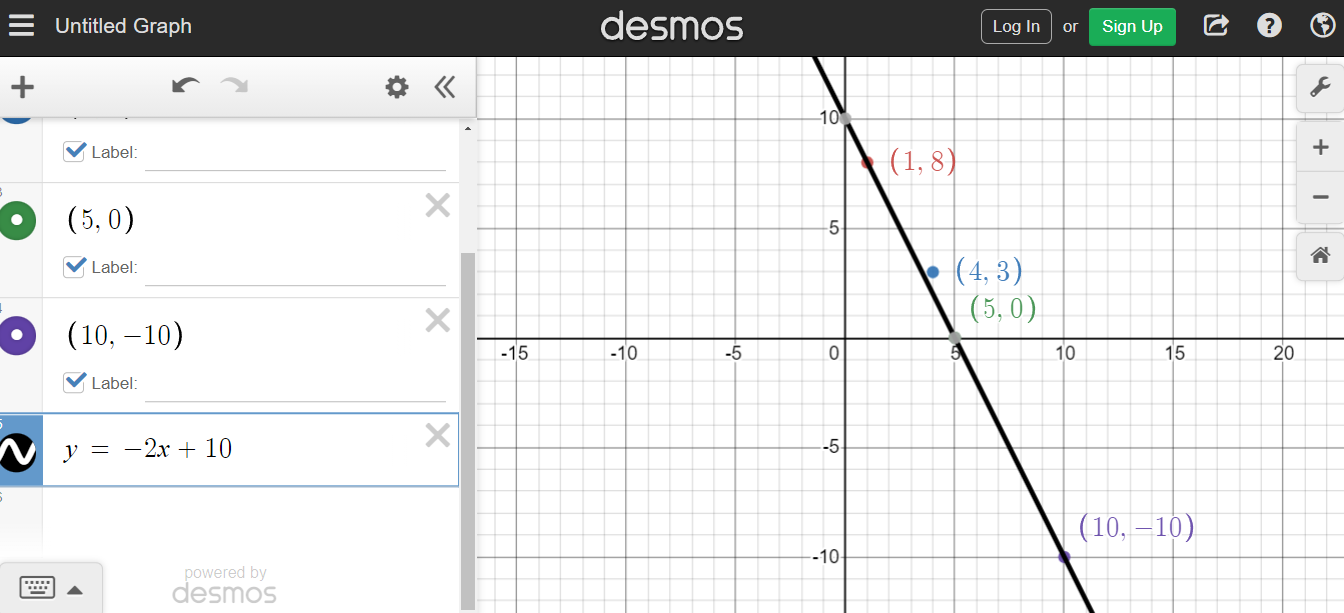Desmos Screenshot

Example Three

I present here a more technical example. Look for how much y goes up by each time x increases by 1. Also the y-intercept is given.

xy
0pi (π)
1
2
3
4

From this table of values the y-intercept is `pi` π. When increases by 1, y increases by 2π. The slope here is 2π.

The equation of the line here is `y = 2πx + π`. Desmos screenshot below.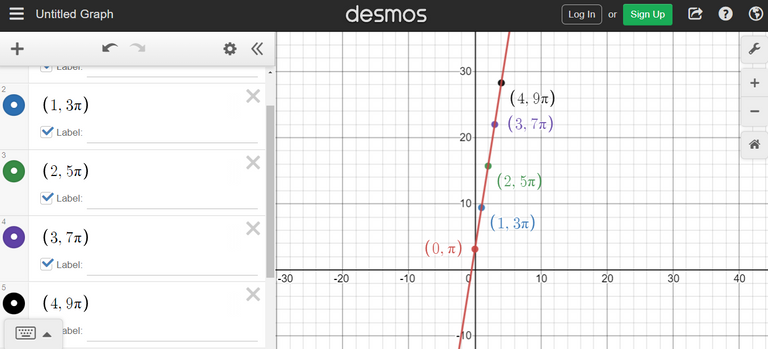Posted with STEMGeeks

Sort:

Fantastic calculation. I love the topic. Math is the simplest course if one understand the rudiments.

Math is the simplest course if one understand the rudiments.

Yes, I do agree with this statement. There are cases where it takes to understand certain technical/abstract concepts. Getting over the hump can be tricky.

10 months ago Reveal Comment
10 months ago Reveal Comment
10 months ago Reveal Comment